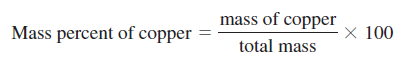# Problem: Sterling silver is a solid solution of silver and copper. If a piece of a sterling silver necklace has a mass of 105.0 g and avolume of 10.12 mL, calculate the mass percent of copper in the piece of necklace. Assume that the volume of silver present plus the volume of copper present equals the total volume. Refer to Table R-5.

⚠️Our tutors found the solution shown to be helpful for the problem you're searching for. We don't have the exact solution yet.

###### Problem Details

Sterling silver is a solid solution of silver and copper. If a piece of a sterling silver necklace has a mass of 105.0 g and a
volume of 10.12 mL, calculate the mass percent of copper in the piece of necklace. Assume that the volume of silver present plus the volume of copper present equals the total volume. Refer to Table R-5.